# Darcy's law

## Darcy's law

Darcy's law is the fundamental law of fluid motion in porous media published by Henry Darcy in 1856 .

## Darcy's law Equation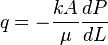$q = -\frac{kA}{\mu} \frac{dP}{dL}$

### Conditions

• Single fluid
• Steady stay flow
• Constant fluid compressibility
• Constant temperature

## Inflow Equations Derivation

Derivation of the Linear and Radial Inflow Equations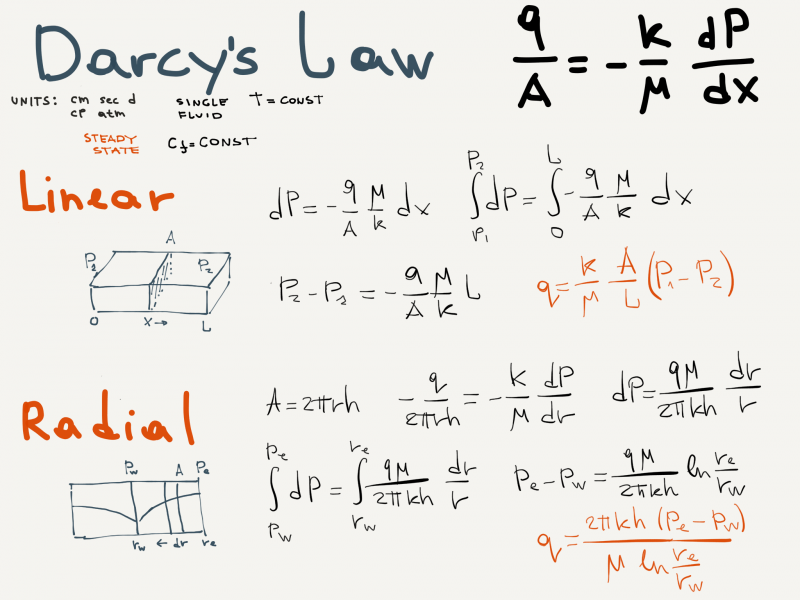## Nomenclature$A$ = cross-sectional area, cm2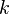$k$ = permeability, d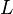$L$ = length, cm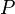$P$ = pressure, atm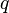$q$ = flow rate, cm3/sec

### Greek symbols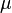$\mu$ = Darcy's law oil viscosity, cp